x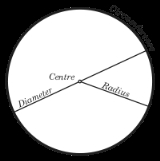CircleOverview

A circle is a simple shape
Shape
The shape of an object located in some space is a geometrical description of the part of that space occupied by the object, as determined by its external boundary – abstracting from location and orientation in space, size, and other properties such as colour, content, and material...

of Euclidean geometry
Euclidean geometry
Euclidean geometry is a mathematical system attributed to the Alexandrian Greek mathematician Euclid, which he described in his textbook on geometry: the Elements. Euclid's method consists in assuming a small set of intuitively appealing axioms, and deducing many other propositions from these...

consisting of those points
Point (geometry)
In geometry, topology and related branches of mathematics a spatial point is a primitive notion upon which other concepts may be defined. In geometry, points are zero-dimensional; i.e., they do not have volume, area, length, or any other higher-dimensional analogue. In branches of mathematics...

in a plane
Plane (mathematics)
In mathematics, a plane is a flat, two-dimensional surface. A plane is the two dimensional analogue of a point , a line and a space...

that are a given distance from a given point, the centre
Centre (geometry)
In geometry, the centre of an object is a point in some sense in the middle of the object. If geometry is regarded as the study of isometry groups then the centre is a fixed point of the isometries.-Circles:...

. The distance between any of the points and the centre is called the radius
Radius
In classical geometry, a radius of a circle or sphere is any line segment from its center to its perimeter. By extension, the radius of a circle or sphere is the length of any such segment, which is half the diameter. If the object does not have an obvious center, the term may refer to its...

.

Circles are simple closed curve
Curve
In mathematics, a curve is, generally speaking, an object similar to a line but which is not required to be straight...

s which divide the plane
Plane (mathematics)
In mathematics, a plane is a flat, two-dimensional surface. A plane is the two dimensional analogue of a point , a line and a space...

into two regions: an interior
Interior (topology)
In mathematics, specifically in topology, the interior of a set S of points of a topological space consists of all points of S that do not belong to the boundary of S. A point that is in the interior of S is an interior point of S....

and an exterior. In everyday use, the term "circle" may be used interchangeably to refer to either the boundary of the figure, or to the whole figure including its interior; in strict technical usage, the circle is the former and the latter is called a disk
Disk (mathematics)
In geometry, a disk is the region in a plane bounded by a circle.A disk is said to be closed or open according to whether or not it contains the circle that constitutes its boundary...

.

A circle is a special ellipse
Ellipse
In geometry, an ellipse is a plane curve that results from the intersection of a cone by a plane in a way that produces a closed curve. Circles are special cases of ellipses, obtained when the cutting plane is orthogonal to the cone's axis...

in which the two foci
Focus (geometry)
In geometry, the foci are a pair of special points with reference to which any of a variety of curves is constructed. For example, foci can be used in defining conic sections, the four types of which are the circle, ellipse, parabola, and hyperbola...

are coincident and the eccentricity
Eccentricity (mathematics)
In mathematics, the eccentricity, denoted e or \varepsilon, is a parameter associated with every conic section. It can be thought of as a measure of how much the conic section deviates from being circular.In particular,...

is 0.DiscussionsEccentricity of the circleEccentricity of the circleMy question is how to define the eccentricity of the circle????????? i know that the eccentricity of the circle is 0. but i am confused on the way i...Encyclopedia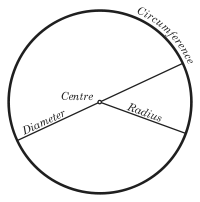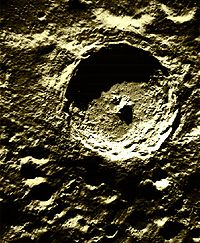A circle is a simple shape
Shape
The shape of an object located in some space is a geometrical description of the part of that space occupied by the object, as determined by its external boundary – abstracting from location and orientation in space, size, and other properties such as colour, content, and material...

of Euclidean geometry
Euclidean geometry
Euclidean geometry is a mathematical system attributed to the Alexandrian Greek mathematician Euclid, which he described in his textbook on geometry: the Elements. Euclid's method consists in assuming a small set of intuitively appealing axioms, and deducing many other propositions from these...

consisting of those points
Point (geometry)
In geometry, topology and related branches of mathematics a spatial point is a primitive notion upon which other concepts may be defined. In geometry, points are zero-dimensional; i.e., they do not have volume, area, length, or any other higher-dimensional analogue. In branches of mathematics...

in a plane
Plane (mathematics)
In mathematics, a plane is a flat, two-dimensional surface. A plane is the two dimensional analogue of a point , a line and a space...

that are a given distance from a given point, the centre
Centre (geometry)
In geometry, the centre of an object is a point in some sense in the middle of the object. If geometry is regarded as the study of isometry groups then the centre is a fixed point of the isometries.-Circles:...

. The distance between any of the points and the centre is called the radius
Radius
In classical geometry, a radius of a circle or sphere is any line segment from its center to its perimeter. By extension, the radius of a circle or sphere is the length of any such segment, which is half the diameter. If the object does not have an obvious center, the term may refer to its...

.

Circles are simple closed curve
Curve
In mathematics, a curve is, generally speaking, an object similar to a line but which is not required to be straight...

s which divide the plane
Plane (mathematics)
In mathematics, a plane is a flat, two-dimensional surface. A plane is the two dimensional analogue of a point , a line and a space...

into two regions: an interior
Interior (topology)
In mathematics, specifically in topology, the interior of a set S of points of a topological space consists of all points of S that do not belong to the boundary of S. A point that is in the interior of S is an interior point of S....

and an exterior. In everyday use, the term "circle" may be used interchangeably to refer to either the boundary of the figure, or to the whole figure including its interior; in strict technical usage, the circle is the former and the latter is called a disk
Disk (mathematics)
In geometry, a disk is the region in a plane bounded by a circle.A disk is said to be closed or open according to whether or not it contains the circle that constitutes its boundary...

.

A circle is a special ellipse
Ellipse
In geometry, an ellipse is a plane curve that results from the intersection of a cone by a plane in a way that produces a closed curve. Circles are special cases of ellipses, obtained when the cutting plane is orthogonal to the cone's axis...

in which the two foci
Focus (geometry)
In geometry, the foci are a pair of special points with reference to which any of a variety of curves is constructed. For example, foci can be used in defining conic sections, the four types of which are the circle, ellipse, parabola, and hyperbola...

are coincident and the eccentricity
Eccentricity (mathematics)
In mathematics, the eccentricity, denoted e or \varepsilon, is a parameter associated with every conic section. It can be thought of as a measure of how much the conic section deviates from being circular.In particular,...

is 0. Circles are conic section
Conic section
In mathematics, a conic section is a curve obtained by intersecting a cone with a plane. In analytic geometry, a conic may be defined as a plane algebraic curve of degree 2...

s attained when a right circular cone
Conical surface
In geometry, a conical surface is the unbounded surface formed by the union of all the straight lines that pass through a fixed point — the apex or vertex — and any point of some fixed space curve — the directrix — that does not contain the apex...

is intersected by a plane perpendicular to the axis of the cone.

A circle can also be defined as the curve traced out by a point that moves so that its distance from a given point is constant.

## Terminology

A circle's diameter
Diameter
In geometry, a diameter of a circle is any straight line segment that passes through the center of the circle and whose endpoints are on the circle. The diameters are the longest chords of the circle...

is the length of a line segment whose endpoint
Endpoint
An endpoint or end point is a mark of termination or completion.* In geometry, an endpoint is a point at which a line segment or ray terminates* Endpoint , the conclusion of a chemical reaction, particularly for titration...

s lie on the circle and which passes through the centre. This is the largest distance between any two points on the circle. The diameter of a circle is twice the radius
Radius
In classical geometry, a radius of a circle or sphere is any line segment from its center to its perimeter. By extension, the radius of a circle or sphere is the length of any such segment, which is half the diameter. If the object does not have an obvious center, the term may refer to its...

, or distance from the centre to the circle's boundary. The terms "diameter" and "radius" also refer to the line segments which fit these descriptions. The circumference
Circumference
The circumference is the distance around a closed curve. Circumference is a special perimeter.-Circumference of a circle:The circumference of a circle is the length around it....

is the distance around the outside of a circle.

A chord
Chord (geometry)
A chord of a circle is a geometric line segment whose endpoints both lie on the circumference of the circle.A secant or a secant line is the line extension of a chord. More generally, a chord is a line segment joining two points on any curve, such as but not limited to an ellipse...

is a line segment whose endpoints lie on the circle. A diameter is the longest chord in a circle. A tangent
Tangent
In geometry, the tangent line to a plane curve at a given point is the straight line that "just touches" the curve at that point. More precisely, a straight line is said to be a tangent of a curve at a point on the curve if the line passes through the point on the curve and has slope where f...

to a circle is a straight line that touches the circle at a single point, while a secant
Secant line
A secant line of a curve is a line that intersects two points on the curve. The word secant comes from the Latin secare, to cut.It can be used to approximate the tangent to a curve, at some point P...

is an extended chord: a straight line cutting the circle at two points.

An arc
Arc (geometry)
In geometry, an arc is a closed segment of a differentiable curve in the two-dimensional plane; for example, a circular arc is a segment of the circumference of a circle...

of a circle is any connected
Connected space
In topology and related branches of mathematics, a connected space is a topological space that cannot be represented as the union of two or more disjoint nonempty open subsets. Connectedness is one of the principal topological properties that is used to distinguish topological spaces...

part of the circle's circumference. A sector
Circular sector
A circular sector or circle sector, is the portion of a disk enclosed by two radii and an arc, where the smaller area is known as the minor sector and the larger being the major sector. In the diagram, θ is the central angle in radians, r the radius of the circle, and L is the arc length of the...

is a region bounded by two radii and an arc lying between the radii, and a segment
Circular segment
In geometry, a circular segment is an area of a circle informally defined as an area which is "cut off" from the rest of the circle by a secant or a chord. The circle segment constitutes the part between the secant and an arc, excluding the circle's center...

is a region bounded by a chord and an arc lying between the chord's endpoints.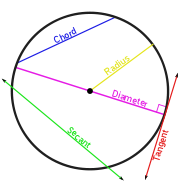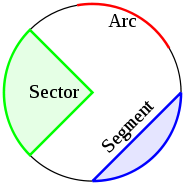## History

The word "circle" derives from the Greek, kirkos "a circle," from the base ker- which means to turn or bend. The origins of the words "circus
Circus
A circus is commonly a travelling company of performers that may include clowns, acrobats, trained animals, trapeze acts, musicians, hoopers, tightrope walkers, jugglers, unicyclists and other stunt-oriented artists...

" and "circuit" are closely related.

The circle has been known since before the beginning of recorded history. Natural circles would have been observed, such as the Moon, Sun, and a short plant stalk blowing in the wind on sand, which forms a circle shape in the sand. The circle is the basis for the wheel
Wheel
A wheel is a device that allows heavy objects to be moved easily through rotating on an axle through its center, facilitating movement or transportation while supporting a load, or performing labor in machines. Common examples found in transport applications. A wheel, together with an axle,...

, which, with related inventions such as gear
Gear
A gear is a rotating machine part having cut teeth, or cogs, which mesh with another toothed part in order to transmit torque. Two or more gears working in tandem are called a transmission and can produce a mechanical advantage through a gear ratio and thus may be considered a simple machine....

s, makes much of modern civilization possible. In mathematics, the study of the circle has helped inspire the development of geometry, astronomy
Astronomy
Astronomy is a natural science that deals with the study of celestial objects and phenomena that originate outside the atmosphere of Earth...

, and calculus.

Early science
Science
Science is a systematic enterprise that builds and organizes knowledge in the form of testable explanations and predictions about the universe...

, particularly geometry
Geometry
Geometry arose as the field of knowledge dealing with spatial relationships. Geometry was one of the two fields of pre-modern mathematics, the other being the study of numbers ....

and astrology and astronomy
Astrology and astronomy
Astrology and astronomy were archaically one and the same discipline , and were only gradually recognized as separate in Western 17th century philosophy ....

, was connected to the divine for most medieval scholars, and many believed that there was something intrinsically "divine" or "perfect" that could be found in circles.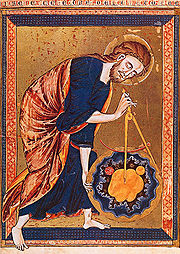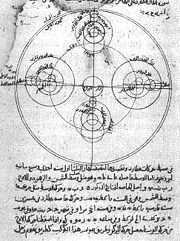Some highlights in the history of the circle are:
• 1700 BC – The Rhind papyrus gives a method to find the area of a circular field. The result corresponds to 256/81 (3.16049...) as an approximate value of .
• 300 BC – Book 3 of Euclid's Elements
Euclid's Elements
Euclid's Elements is a mathematical and geometric treatise consisting of 13 books written by the Greek mathematician Euclid in Alexandria c. 300 BC. It is a collection of definitions, postulates , propositions , and mathematical proofs of the propositions...

deals with the properties of circles.
• In Plato
Plato
Plato , was a Classical Greek philosopher, mathematician, student of Socrates, writer of philosophical dialogues, and founder of the Academy in Athens, the first institution of higher learning in the Western world. Along with his mentor, Socrates, and his student, Aristotle, Plato helped to lay the...

's Seventh Letter there is a detailed definition and explanation of the circle. Plato explains the perfect circle, and how it is different from any drawing, words, definition or explanation.
• 1880 – Lindemann
Ferdinand von Lindemann
Carl Louis Ferdinand von Lindemann was a German mathematician, noted for his proof, published in 1882, that π is a transcendental number, i.e., it is not a root of any polynomial with rational coefficients....

proves that is transcendental, effectively settling the millennia-old problem of squaring the circle
Squaring the circle
Squaring the circle is a problem proposed by ancient geometers. It is the challenge of constructing a square with the same area as a given circle by using only a finite number of steps with compass and straightedge...

.

### Length of circumference

The ratio of a circle's circumference
Circumference
The circumference is the distance around a closed curve. Circumference is a special perimeter.-Circumference of a circle:The circumference of a circle is the length around it....

to its diameter
Diameter
In geometry, a diameter of a circle is any straight line segment that passes through the center of the circle and whose endpoints are on the circle. The diameters are the longest chords of the circle...

is
Pi
' is a mathematical constant that is the ratio of any circle's circumference to its diameter. is approximately equal to 3.14. Many formulae in mathematics, science, and engineering involve , which makes it one of the most important mathematical constants...

(pi), an irrational
Irrational number
In mathematics, an irrational number is any real number that cannot be expressed as a ratio a/b, where a and b are integers, with b non-zero, and is therefore not a rational number....

constant
Mathematical constant
A mathematical constant is a special number, usually a real number, that is "significantly interesting in some way". Constants arise in many different areas of mathematics, with constants such as and occurring in such diverse contexts as geometry, number theory and calculus.What it means for a...

approximately equal to 3.141592654. Thus the length of the circumference is related to the radius and diameter by: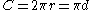### Area enclosed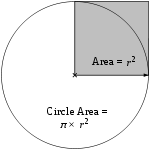As proved by Archimedes, the area enclosed by a circle is equal to that of a triangle whose base has the length of the circle's circumference and whose height equals the circle's radius, which comes to multiplied by the radius squared: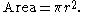Equivalently, denoting diameter by ,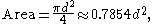that is, approximately 79 percent of the circumscribing square (whose side is of length ).

The circle is the plane curve enclosing the maximum area for a given arc length. This relates the circle to a problem in the calculus of variations
Calculus of variations
Calculus of variations is a field of mathematics that deals with extremizing functionals, as opposed to ordinary calculus which deals with functions. A functional is usually a mapping from a set of functions to the real numbers. Functionals are often formed as definite integrals involving unknown...

, namely the isoperimetric inequality.

#### Cartesian coordinatesIn an - Cartesian coordinate system
Cartesian coordinate system
A Cartesian coordinate system specifies each point uniquely in a plane by a pair of numerical coordinates, which are the signed distances from the point to two fixed perpendicular directed lines, measured in the same unit of length...

, the circle with centre coordinates
Coordinate system
In geometry, a coordinate system is a system which uses one or more numbers, or coordinates, to uniquely determine the position of a point or other geometric element. The order of the coordinates is significant and they are sometimes identified by their position in an ordered tuple and sometimes by...

and radius is the set of all points such that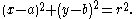This equation
Equation
An equation is a mathematical statement that asserts the equality of two expressions. In modern notation, this is written by placing the expressions on either side of an equals sign , for examplex + 3 = 5\,asserts that x+3 is equal to 5...

of the circle follows from the Pythagorean theorem
Pythagorean theorem
In mathematics, the Pythagorean theorem or Pythagoras' theorem is a relation in Euclidean geometry among the three sides of a right triangle...

applied to any point on the circle: as shown in the diagram to the right, the radius is the hypotenuse of a right-angled triangle whose other sides are of length  −  and  − . If the circle is centred at the origin (0, 0), then the equation simplifies to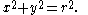The equation can be written in parametric form using the trigonometric function
Trigonometric function
In mathematics, the trigonometric functions are functions of an angle. They are used to relate the angles of a triangle to the lengths of the sides of a triangle...

s sine and cosine as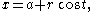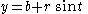where t is a parametric variable in the range 0 to 2π, interpreted geometrically as the angle that the ray from to makes with the -axis. An alternative parametrisation of the circle is: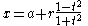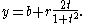In this parametrisation, the ratio of to can be interpreted geometrically as the stereographic projection
Stereographic projection
The stereographic projection, in geometry, is a particular mapping that projects a sphere onto a plane. The projection is defined on the entire sphere, except at one point — the projection point. Where it is defined, the mapping is smooth and bijective. It is conformal, meaning that it...

of the circle onto the line passing through the centre parallel to the -axis.

In homogeneous coordinates
Homogeneous coordinates
In mathematics, homogeneous coordinates, introduced by August Ferdinand Möbius in his 1827 work Der barycentrische Calcül, are a system of coordinates used in projective geometry much as Cartesian coordinates are used in Euclidean geometry. They have the advantage that the coordinates of points,...

each conic section
Conic section
In mathematics, a conic section is a curve obtained by intersecting a cone with a plane. In analytic geometry, a conic may be defined as a plane algebraic curve of degree 2...

with equation of a circle is of the form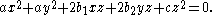It can be proven that a conic section is a circle exactly when it contains (when extended to the complex projective plane) the points I(1: i: 0) and J(1: −i: 0). These points are called the circular points at infinity
Circular points at infinity
In projective geometry, the circular points at infinity in the complex projective plane are and ....

.

#### Polar coordinates

In polar coordinates the equation of a circle is: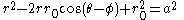where is the radius of the circle, 0 is the distance from the origin to the centre of the circle, and φ is the anticlockwise angle from the positive -axis to the line connecting the origin to the centre of the circle. For a circle centred at the origin, i.e. 0 = 0, this reduces to simply = . When 0 = , or when the origin lies on the circle, the equation becomes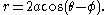In the general case, the equation can be solved for , giving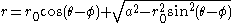,
the solution with a minus sign in front of the square root giving the same curve.

#### Complex plane

In the complex plane
Complex plane
In mathematics, the complex plane or z-plane is a geometric representation of the complex numbers established by the real axis and the orthogonal imaginary axis...

, a circle with a centre at and radius has the equation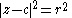. In parametric form this can be written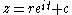.

The slightly generalised equation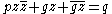for real , and complex is sometimes called a generalised circle
Generalised circle
A generalized circle, also referred to as a "cline" or "circline", is a straight line or a circle. The concept is mainly used in inversive geometry, because straight lines and circles have very similar properties in that geometry and are best treated together....

. This becomes the above equation for a circle with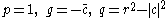, since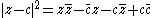. Not all generalised circles are actually circles: a generalised circle is either a (true) circle or a line
Line (geometry)
The notion of line or straight line was introduced by the ancient mathematicians to represent straight objects with negligible width and depth. Lines are an idealization of such objects...

.

### Tangent lines

The tangent line through a point on the circle is perpendicular to the diameter passing through . If = (1, 1) and the circle has centre and radius , then the tangent line is perpendicular to the line from to (1,1), so it has the form (1−)+(1−) = . Evaluating at (1, 1) determines the value of and the result is that the equation of the tangent is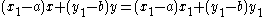or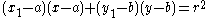.

If 1≠ then slope of this line is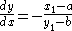.
This can also be found using implicit differentiation.

When the centre of the circle is at the origin then the equation of the tangent line becomes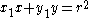,
and its slope is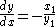.

## Properties

• The circle is the shape with the largest area for a given length of perimeter. (See Isoperimetric inequality.)
• The circle is a highly symmetric shape: every line through the centre forms a line of reflection symmetry
Reflection symmetry
Reflection symmetry, reflectional symmetry, line symmetry, mirror symmetry, mirror-image symmetry, or bilateral symmetry is symmetry with respect to reflection. That is, a figure which does not change upon undergoing a reflection has reflectional symmetry.In 2D there is a line of symmetry, in 3D a...

and it has rotational symmetry
Rotational symmetry
Generally speaking, an object with rotational symmetry is an object that looks the same after a certain amount of rotation. An object may have more than one rotational symmetry; for instance, if reflections or turning it over are not counted, the triskelion appearing on the Isle of Man's flag has...

around the centre for every angle. Its symmetry group
Symmetry group
The symmetry group of an object is the group of all isometries under which it is invariant with composition as the operation...

is the orthogonal group
Orthogonal group
In mathematics, the orthogonal group of degree n over a field F is the group of n × n orthogonal matrices with entries from F, with the group operation of matrix multiplication...

(2,). The group of rotations alone is the circle group .
• All circles are similar
Similarity (geometry)
Two geometrical objects are called similar if they both have the same shape. More precisely, either one is congruent to the result of a uniform scaling of the other...

.
• A circle's circumference and radius are proportional
Proportionality (mathematics)
In mathematics, two variable quantities are proportional if one of them is always the product of the other and a constant quantity, called the coefficient of proportionality or proportionality constant. In other words, are proportional if the ratio \tfrac yx is constant. We also say that one...

.
• The area enclosed and the square of its radius are proportional
Proportionality (mathematics)
In mathematics, two variable quantities are proportional if one of them is always the product of the other and a constant quantity, called the coefficient of proportionality or proportionality constant. In other words, are proportional if the ratio \tfrac yx is constant. We also say that one...

.
• The constants
Mathematical constant
A mathematical constant is a special number, usually a real number, that is "significantly interesting in some way". Constants arise in many different areas of mathematics, with constants such as and occurring in such diverse contexts as geometry, number theory and calculus.What it means for a...

of proportionality are 2 and , respectively.
• The circle which is centred at the origin with radius 1 is called the unit circle
Unit circle
In mathematics, a unit circle is a circle with a radius of one. Frequently, especially in trigonometry, "the" unit circle is the circle of radius one centered at the origin in the Cartesian coordinate system in the Euclidean plane...

.
• Thought of as a great circle
Great circle
A great circle, also known as a Riemannian circle, of a sphere is the intersection of the sphere and a plane which passes through the center point of the sphere, as opposed to a general circle of a sphere where the plane is not required to pass through the center...

of the unit sphere
Unit sphere
In mathematics, a unit sphere is the set of points of distance 1 from a fixed central point, where a generalized concept of distance may be used; a closed unit ball is the set of points of distance less than or equal to 1 from a fixed central point...

, it becomes the Riemannian circle
Riemannian circle
In metric space theory and Riemannian geometry, the Riemannian circle is a great circle equipped with its great-circle distance...

.
• Through any three points, not all on the same line, there lies a unique circle. In Cartesian coordinates, it is possible to give explicit formulae for the coordinates of the centre of the circle and the radius in terms of the coordinates of the three given points. See circumcircle.

### Chord

• Chords are equidistant from the centre of a circle if and only if they are equal in length.
• The perpendicular bisector of a chord passes through the centre of a circle; equivalent statements stemming from the uniqueness of the perpendicular bisector:
• A perpendicular line from the centre of a circle bisects the chord.
• The line segment
Line segment
In geometry, a line segment is a part of a line that is bounded by two end points, and contains every point on the line between its end points. Examples of line segments include the sides of a triangle or square. More generally, when the end points are both vertices of a polygon, the line segment...

(circular segment
Circular segment
In geometry, a circular segment is an area of a circle informally defined as an area which is "cut off" from the rest of the circle by a secant or a chord. The circle segment constitutes the part between the secant and an arc, excluding the circle's center...

) through the centre bisecting a chord is perpendicular to the chord.
• If a central angle and an inscribed angle
Inscribed angle
In geometry, an inscribed angle is formed when two secant lines of a circle intersect on the circle....

of a circle are subtended by the same chord and on the same side of the chord, then the central angle is twice the inscribed angle.
• If two angles are inscribed on the same chord and on the same side of the chord, then they are equal.
• If two angles are inscribed on the same chord and on opposite sides of the chord, then they are supplemental.
• For a cyclic quadrilateral, the exterior angle is equal to the interior opposite angle.
• An inscribed angle subtended by a diameter is a right angle (see Thales' theorem
Thales' theorem
In geometry, Thales' theorem states that if A, B and C are points on a circle where the line AC is a diameter of the circle, then the angle ABC is a right angle. Thales' theorem is a special case of the inscribed angle theorem...

).
• The diameter is the longest chord of the circle.
• If the intersection of any two chords divides one chord into lengths and and divides the other chord into lengths and , then = .
• If the intersection of any two perpendicular chords divides one chord into lengths and and divides the other chord into lengths and , then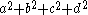equals the square of the diameter.
• The distance from a point on the circle to a given chord times the diameter of the circle equals the product of the distances from the point to the ends of the chord.

### Sagitta

• The sagitta (also known as the versine
Versine
The versine or versed sine, versin, is a trigonometric function equal to and 2sin2. It appeared in some of the earliest trigonometric tables and was once widespread, but it is now little-used...

) is a line segment drawn perpendicular to a chord, between the midpoint of that chord and the arc of the circle.
• Given the length of a chord, and the length of the sagitta, the Pythagorean theorem
Pythagorean theorem
In mathematics, the Pythagorean theorem or Pythagoras' theorem is a relation in Euclidean geometry among the three sides of a right triangle...

can be used to calculate the radius of the unique circle which will fit around the two lines: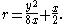Another proof of this result which relies only on two chord properties given above is as follows. Given a chord of length and with sagitta of length , since the sagitta intersects the midpoint of the chord, we know it is part of a diameter of the circle. Since the diameter is twice the radius, the “missing” part of the diameter is (2 − ) in length. Using the fact that one part of one chord times the other part is equal to the same product taken along a chord intersecting the first chord, we find that (2 − ) = (/2)2. Solving for , we find the required result.

### Tangent

• The line perpendicular drawn to a radius through the end point of the radius is a tangent to the circle.
• A line drawn perpendicular to a tangent through the point of contact with a circle passes through the centre of the circle.
• Two tangents can always be drawn to a circle from any point outside the circle, and these tangents are equal in length.
• If a tangent at A and a tangent at B intersect at the exterior point , then denoting the centre as , the angles ∠ and ∠ are supplementary
Supplementary angles
Supplementary angles are pairs of angles that add up to 180 degrees. Thus the supplement of an angle of x degrees is an angle of degrees....

.
• If is tangent to the circle at and if is a chord of the circle, then ∠ =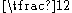arc.

### Theorems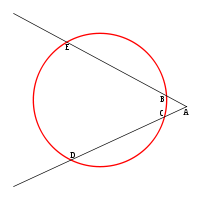• The chord theorem states that if two chords, and , intersect at , then × = ×.
• If a tangent
Tangent
In geometry, the tangent line to a plane curve at a given point is the straight line that "just touches" the curve at that point. More precisely, a straight line is said to be a tangent of a curve at a point on the curve if the line passes through the point on the curve and has slope where f...

from an external point meets the circle at and a secant
Secant line
A secant line of a curve is a line that intersects two points on the curve. The word secant comes from the Latin secare, to cut.It can be used to approximate the tangent to a curve, at some point P...

from the external point meets the circle at and respectively, then 2 = ×. (Tangent-secant theorem.)
• If two secants, and , also cut the circle at and respectively, then × = ×. (Corollary of the tangent-secant theorem.)
• The angle between a tangent and chord is equal to one half the subtended angle on the opposite side of the chord (Tangent Chord Angle).
• If the angle subtended by the chord at the centre is 90 degrees
Degree (angle)
A degree , usually denoted by ° , is a measurement of plane angle, representing 1⁄360 of a full rotation; one degree is equivalent to π/180 radians...

then = √2 × , where is the length of the chord and is the radius of the circle.
• If two secants are inscribed in the circle as shown at right, then the measurement of angle is equal to one half the difference of the measurements of the enclosed arcs ( and ). This is the secant-secant theorem.

### Inscribed angles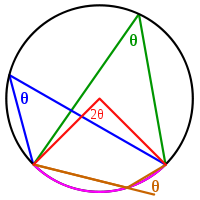An inscribed angle
Inscribed angle
In geometry, an inscribed angle is formed when two secant lines of a circle intersect on the circle....

(examples are the blue and green angles in the figure) is exactly half the corresponding central angle
Central angle
A central angle is an angle which vertex is the center of a circle, and whose sides pass through a pair of points on the circle, thereby subtending an arc between those two points whose angle is equal to the central angle itself...

(red). Hence, all inscribed angles that subtend the same arc (pink) are equal. Angles inscribed on the arc (brown) are supplementary. In particular, every inscribed angle that subtends a diameter
Diameter
In geometry, a diameter of a circle is any straight line segment that passes through the center of the circle and whose endpoints are on the circle. The diameters are the longest chords of the circle...

is a right angle
Right angle
In geometry and trigonometry, a right angle is an angle that bisects the angle formed by two halves of a straight line. More precisely, if a ray is placed so that its endpoint is on a line and the adjacent angles are equal, then they are right angles...

(since the central angle is 180 degrees).

## Circle of Apollonius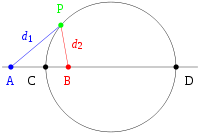Apollonius of Perga
Apollonius of Perga
Apollonius of Perga [Pergaeus] was a Greek geometer and astronomer noted for his writings on conic sections. His innovative methodology and terminology, especially in the field of conics, influenced many later scholars including Ptolemy, Francesco Maurolico, Isaac Newton, and René Descartes...

showed that a circle may also be defined as the set of points in a plane having a constant ratio (other than 1) of distances to two fixed foci, and . (The set of points where the distances are equal is the perpendicular bisector of and , a line.) That circle is sometimes said to be drawn about two points.

The proof is in two parts. First, one must prove that, given two foci and and a ratio of distances, any point satisfying the ratio of distances must fall on a particular circle. Let be another point, also satisfying the ratio and lying on segment . By the angle bisector theorem
Angle bisector theorem
In geometry, the angle bisector theorem is concerned with the relative lengths of the two segments that a triangle's side is divided into by a line that bisects the opposite angle. It equates their relative lengths to the relative lengths of the other two sides of the triangle.Consider a triangle...

the line segment will bisect the interior angle , since the segments are similar: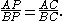Analogously, a line segment through some point on extended bisects the corresponding exterior angle  where is on extended. Since the interior and exterior angles sum to 180 degrees, the angle CPD is exactly 90 degrees, i.e., a right angle
Right angle
In geometry and trigonometry, a right angle is an angle that bisects the angle formed by two halves of a straight line. More precisely, if a ray is placed so that its endpoint is on a line and the adjacent angles are equal, then they are right angles...

. The set of points such that angle is a right angle forms a circle, of which is a diameter.

Second, see for a proof that every point on the indicated circle satisfies the given ratio.

### Cross-ratios

A closely related property of circles involves the geometry of the cross-ratio
Cross-ratio
In geometry, the cross-ratio, also called double ratio and anharmonic ratio, is a special number associated with an ordered quadruple of collinear points, particularly points on a projective line...

of points in the complex plane
Complex plane
In mathematics, the complex plane or z-plane is a geometric representation of the complex numbers established by the real axis and the orthogonal imaginary axis...

. If , , and are as above, then the circle of Apollonius for these three points is the collection of points for which the absolute value of the cross-ratio is equal to one: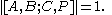Stated another way, is a point on the circle of Apollonius if and only if the cross-ratio [,;,] is on the unit circle
Unit circle
In mathematics, a unit circle is a circle with a radius of one. Frequently, especially in trigonometry, "the" unit circle is the circle of radius one centered at the origin in the Cartesian coordinate system in the Euclidean plane...

in the complex plane.

### Generalized circles

If is the midpoint
Midpoint
The midpoint is the middle point of a line segment. It is equidistant from both endpoints.-Formulas:...

of the segment , then the collection of points satisfying the Apollonius condition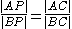(1)

is not a circle, but rather a line.

Thus, if , , and are given distinct points in the plane, then the locus of points satisfying (1) is called a generalized circle. It may either be a true circle or a line. In this sense a line
Line (geometry)
The notion of line or straight line was introduced by the ancient mathematicians to represent straight objects with negligible width and depth. Lines are an idealization of such objects...

is a generalized circle of infinite radius.

## Circles inscribed in or circumscribed about other figures

In every triangle
Triangle
A triangle is one of the basic shapes of geometry: a polygon with three corners or vertices and three sides or edges which are line segments. A triangle with vertices A, B, and C is denoted ....

a unique circle, called the incircle
Incircle and excircles of a triangle
In geometry, the incircle or inscribed circle of a triangle is the largest circle contained in the triangle; it touches the three sides...

, can be inscribed such that it is tangent
Tangent
In geometry, the tangent line to a plane curve at a given point is the straight line that "just touches" the curve at that point. More precisely, a straight line is said to be a tangent of a curve at a point on the curve if the line passes through the point on the curve and has slope where f...

to each of the three sides of the triangle.

About every triangle a unique circle, called the circumcircle, can be circumscribed such that it goes through each of the triangle's three vertices
Vertex (geometry)
In geometry, a vertex is a special kind of point that describes the corners or intersections of geometric shapes.-Of an angle:...

.

A tangential polygon, such as a tangential quadrilateral
Tangential quadrilateral
In Euclidean geometry, a tangential quadrilateral or circumscribed quadrilateral is a convex quadrilateral whose sides all lie tangent to a single circle inscribed within the quadrilateral. This circle is called the incircle...

, is any convex polygon
Convex polygon
In geometry, a polygon can be either convex or concave .- Convex polygons :A convex polygon is a simple polygon whose interior is a convex set...

within which a circle can be inscribed that is tangent to each side of the polygon.

A cyclic polygon is any convex polygon about which a circle can be circumscribed, passing through each vertex. A well-studied example is the cyclic quadrilateral
Cyclic quadrilateral
In Euclidean geometry, a cyclic quadrilateral is a quadrilateral whose vertices all lie on a single circle. This circle is called the circumcircle or circumscribed circle, and the vertices are said to be concyclic. Other names for these quadrilaterals are chordal quadrilateral and inscribed...

.

A hypocycloid
Hypocycloid
In geometry, a hypocycloid is a special plane curve generated by the trace of a fixed point on a small circle that rolls within a larger circle...

is a curve that is inscribed in a given circle by tracing a fixed point on a smaller circle that rolls within and tangent to the given circle.

## Circle as limiting case of other figures

The circle can be viewed as a limiting case of each of various other figures:
• A Cartesian oval
Cartesian oval
In geometry, a Cartesian oval, named after René Descartes, is determined as follows. Let and be fixed points in the plane, and let and denote the Euclidean distances from these points to a third variable point . Let and be arbitrary real numbers. Then the Cartesian oval is the locus of...

is a set of points such that a weighted sum of the distances from any of its points to two fixed points (foci
Focus (geometry)
In geometry, the foci are a pair of special points with reference to which any of a variety of curves is constructed. For example, foci can be used in defining conic sections, the four types of which are the circle, ellipse, parabola, and hyperbola...

) is a constant. An ellipse
Ellipse
In geometry, an ellipse is a plane curve that results from the intersection of a cone by a plane in a way that produces a closed curve. Circles are special cases of ellipses, obtained when the cutting plane is orthogonal to the cone's axis...

is the case in which the weights are equal. A circle is an ellipse with an eccentricity
Eccentricity (mathematics)
In mathematics, the eccentricity, denoted e or \varepsilon, is a parameter associated with every conic section. It can be thought of as a measure of how much the conic section deviates from being circular.In particular,...

of zero, meaning that the two foci coincide with each other as the centre of the circle. A circle is also a different special case of a Cartesian oval in which one of the weights is zero.

• A superellipse
Superellipse
A superellipse is a geometric figure defined in the Cartesian coordinate system as the set of all points withwhere n, a and b are positive numbers....

has an equation of the form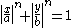for positive , , and . A supercircle has =. A circle is the special case of a supercircle in which =2.

• A Cassini oval
Cassini oval
A Cassini oval is a plane curve defined as the set of points in the plane such that the product of the distances to two fixed points is constant. This is related to an ellipse, for which the...

is a set of points such that the product of the distances from any of its points to two fixed points is a constant. When the two fixed points coincide, a circle results.

• A curve of constant width
Curve of constant width
In geometry, a curve of constant width is a convex planar shape whose width, defined as the perpendicular distance between two distinct parallel lines each intersecting its boundary in a single point, is the same regardless of the direction of those two parallel lines.More generally, any compact...

is a figure whose width, defined as the perpendicular distance between two distinct parallel lines each intersecting its boundary in a single point, is the same regardless of the direction of those two parallel lines. The circle is the simplest example of this type of figure.

## See also

• Affine sphere
Affine sphere
In mathematics, and especially differential geometry, an affine sphere is a hypersurface for which the affine normals all intersect in a single point...

• Annulus (mathematics)
Annulus (mathematics)
In mathematics, an annulus is a ring-shaped geometric figure, or more generally, a term used to name a ring-shaped object. Or, it is the area between two concentric circles...

• List of circle topics
• Loop
Loop
- Technology :*Loop , sending a signal on a channel and receiving it back at the sending terminal*Loop , a method of control flow in computer science*Loop device, a Unix device node that allows a file to be mounted on a directory...

• Sphere
Sphere
A sphere is a perfectly round geometrical object in three-dimensional space, such as the shape of a round ball. Like a circle in two dimensions, a perfect sphere is completely symmetrical around its center, with all points on the surface lying the same distance r from the center point...

## External links

• Circle (PlanetMath.org website)
• Interactive Java applets for the properties of and elementary constructions involving circles.
• Interactive Standard Form Equation of Circle Click and drag points to see standard form equation in action
• Munching on Circles at cut-the-knot
Cut-the-knot
Cut-the-knot is a free, advertisement-funded educational website maintained by Alexander Bogomolny and devoted to popular exposition of many topics in mathematics. The site has won more than 20 awards from scientific and educational publications, including a Scientific American Web Award in 2003,...

• MathAce's Circle article – has a good in-depth explanation of unit circles and transforming circular equations.
The source of this article is wikipedia, the free encyclopedia.  The text of this article is licensed under the GFDL.